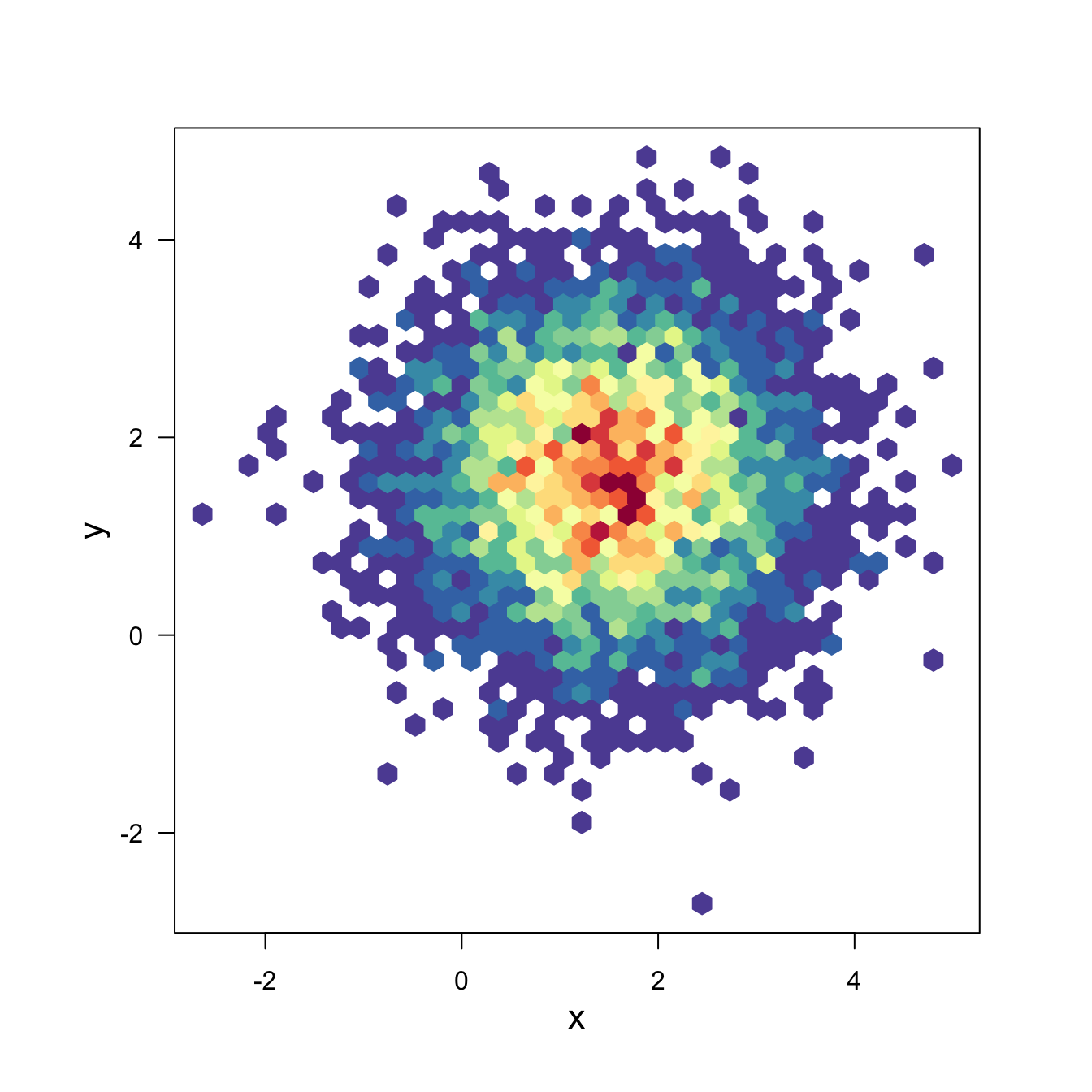# Hexbin chart with the hexbin package

This post explains how to build a hexbin chart with R using the `hexbin` package. Hexbin chart is a 2d density chart, allowing to visualize the relationship between 2 numeric variables.

Scatterplots can get very hard to interpret when displaying large datasets, as points inevitably overplot and can???t be individually discerned.

Binning can be though of as a two-dimensional histogram, where shades of the bins take the place of the heights of the bars. This technique is computed in the `hexbin` package.``````# Packages
library(hexbin)
library(RColorBrewer)

# Create data
x <- rnorm(mean=1.5, 5000)
y <- rnorm(mean=1.6, 5000)

# Make the plot
bin<-hexbin(x, y, xbins=40)
my_colors=colorRampPalette(rev(brewer.pal(11,'Spectral')))
plot(bin, main="" , colramp=my_colors , legend=F ) ``````

Related chart types

## Contact

This document is a work by Yan Holtz. Any feedback is highly encouraged. You can fill an issue on Github, drop me a message on Twitter, or send an email pasting yan.holtz.data with gmail.com.